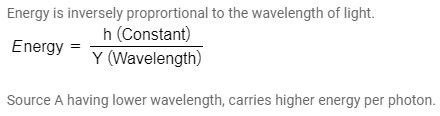# Test: Sources Of Energy - 4

## 25 Questions MCQ Test Science Class 10 | Test: Sources Of Energy - 4

Description
This mock test of Test: Sources Of Energy - 4 for UPSC helps you for every UPSC entrance exam. This contains 25 Multiple Choice Questions for UPSC Test: Sources Of Energy - 4 (mcq) to study with solutions a complete question bank. The solved questions answers in this Test: Sources Of Energy - 4 quiz give you a good mix of easy questions and tough questions. UPSC students definitely take this Test: Sources Of Energy - 4 exercise for a better result in the exam. You can find other Test: Sources Of Energy - 4 extra questions, long questions & short questions for UPSC on EduRev as well by searching above.
QUESTION: 1

Solution:
QUESTION: 2

Solution:
QUESTION: 3

### Which of the following is the potential site for harnessing tidal energy in India ?

Solution:
QUESTION: 4

To work properly, wind-electric generator’s need wind speeds of at least about-

Solution:
QUESTION: 5

Which of the following acts as control rods in Nuclear reactor ?

Solution:

Cadmium rods are used as control rods as they are capable of absorbing neutrons without themselves Fissioning. Boron rods are used as control rods in nuclear  reactor to control the fission rate of uranium and plutonium.

QUESTION: 6

Name any one element which is used in making solar cells.

Solution:
QUESTION: 7

The mass number of an isotope of an element is 295, if its nucleus has 196 neutrons. What is its atomic number ?

Solution:

Atomic Number = Mass no. - No. Of neutrons
⇒ 295 - 196
⇒  99
So, option ( a) is the correct answer.

QUESTION: 8

Most of the sources of energy we use represent stored solar energy. Which of the following is not ultimately derived from the Sun’s energy ?

Solution:
QUESTION: 9

What is the full from of OTEC

Solution:
QUESTION: 10

Statement A : Oceans serve as the most potential source of renewable energy
Statement B : Energy from nuclear fusion creates more pollution than energy from nuclear fission

Solution:
QUESTION: 11

Which of the following factors is responsible for blowing of wind ?

Solution:
QUESTION: 12

What is present contribution of hydro electric power stations to the electricity generated in India ?

Solution:
QUESTION: 13

Which of the following is incorrect statement regarding solar cooker?

Solution:
QUESTION: 14

If the energy associated with 1amu is 931Mev than the energy associated with 3/2 amu is

Solution:

1 amu energy = 931 MeV
Therefore, 3/2 amu = [ 3/2 * 931] MeV
⇒ 1396.5 MeV

*Multiple options can be correct
QUESTION: 15

Which of the following is not present in Hydrogen Bomb.

Solution:

To make a hydrogen bomb, one would still need uranium or plutonium as well as two other isotopes of hydrogen, called deuterium and tritium. The hydrogen bomb relies on fusion, the process of taking two separate atoms and putting them together to form a third atom.

QUESTION: 16

Why do we use black surfaces in solar devices ?

Solution:
QUESTION: 17

Lights from two different sources A and B have wavelength 0.3 micron and 0.7 micron respectively. Which one of the two light carry more energy per photon ?

Solution:QUESTION: 18

Statement A : Oceans serve as the most potential source of renewable energy
Statement B : Energy from nuclear fusion creates more pollution than energy from nuclear fission

Solution:
QUESTION: 19

Which energy is not derived from the sun ?

Solution:
QUESTION: 20

A solar panel is made by combining a large member of-

Solution:
QUESTION: 21

The site of hydroelectric plant should be chosen carefully because it-

Solution:
QUESTION: 22

The conditions for producing biogas is

Solution:

Biogas is produced from organic waste which biodegrades by means of bacteria in an anaerobic environment through a process of Anaerobic Digestion. It uses anaerobic digestion to recycle the organic materials (plant and animal products), turning them into biogas, which contains both energy (gas), and valuable soil products (liquids and solids). Anaerobic digestion is a series of biological processes in which microorganisms break down biodegradable material in the absence of oxygen. Hence the correct answer would be (A).

QUESTION: 23

In addition to visible light, which other type of radiations are emitted by a 100w electric Lamp when it is glowing ?

Solution:
QUESTION: 24

Geothermal energy is feasible in regions that-

Solution:
QUESTION: 25

The mass number of elements P, Q, R are 2, 180, 235 respectively which one of them is most suitable to make a hydrogen bomb ?

Solution:

A hydrogen bomb is based on nuclear fusion reactions. So, a light element P of mass number 2 is the most suitable to make it.